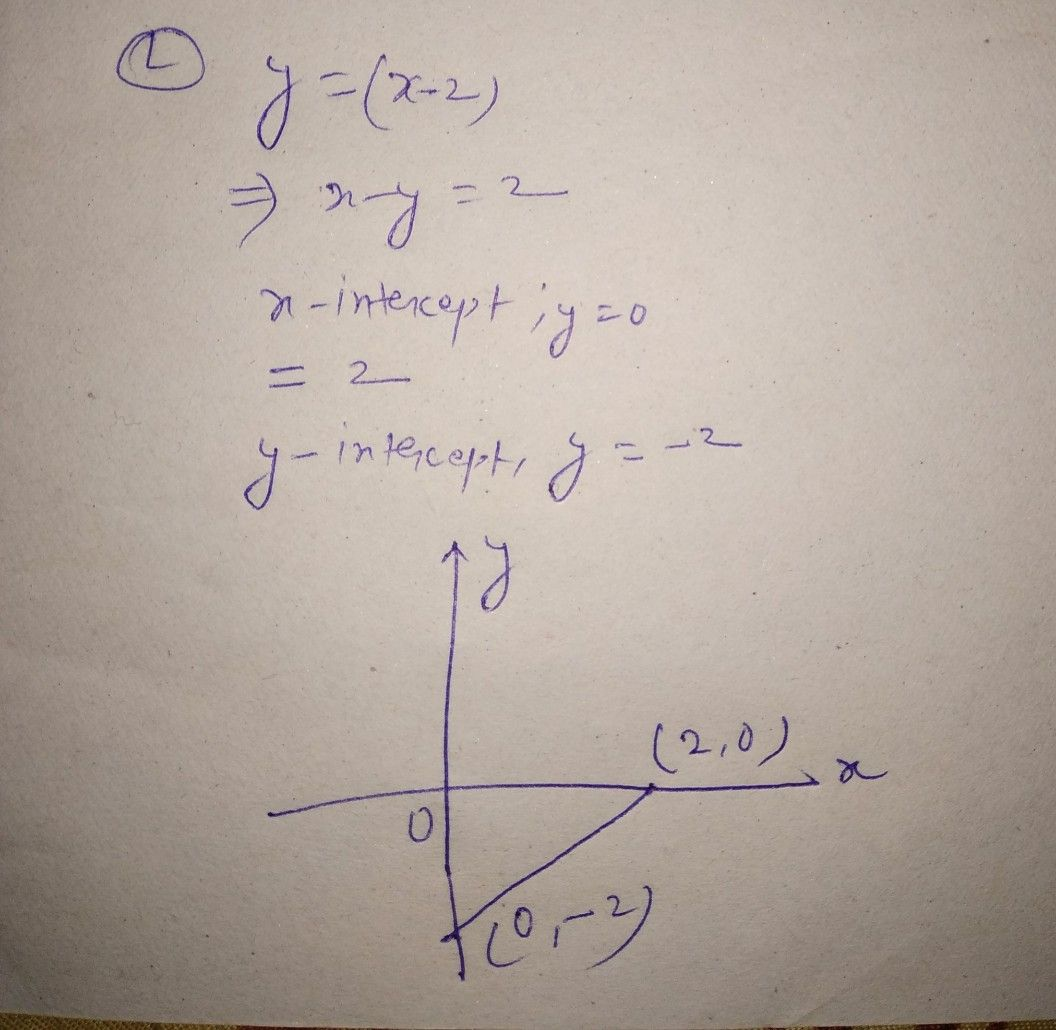Symbol
ProblemGraph the following logarithmic functions using an online graphing calculator then find its domain and range. $1.$ $y=\left(x-2\right)$ $5$ $y=\left(x+1\right)$ $9.y=x+2$ $2.y=\left(x+3\right)$ $6.y=x-2$ $10.y=x+1$ $3.y=\left(x-1\right)$ $7.y=x+3$ $4.y=\left(x+2\right)$ $8$ $y=x-1$
10th-13th grade
Other
Search count: 106
SolutionQanda teacher - jaisifor other question you have to ask separately